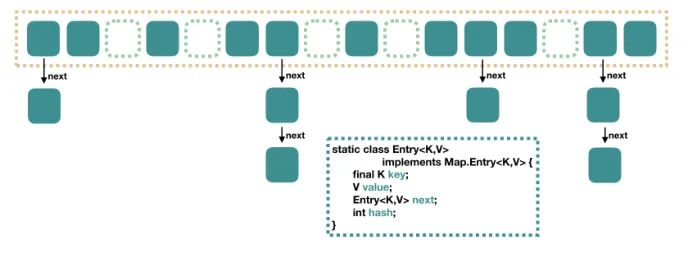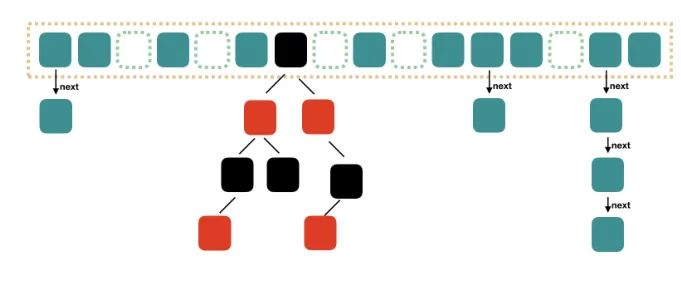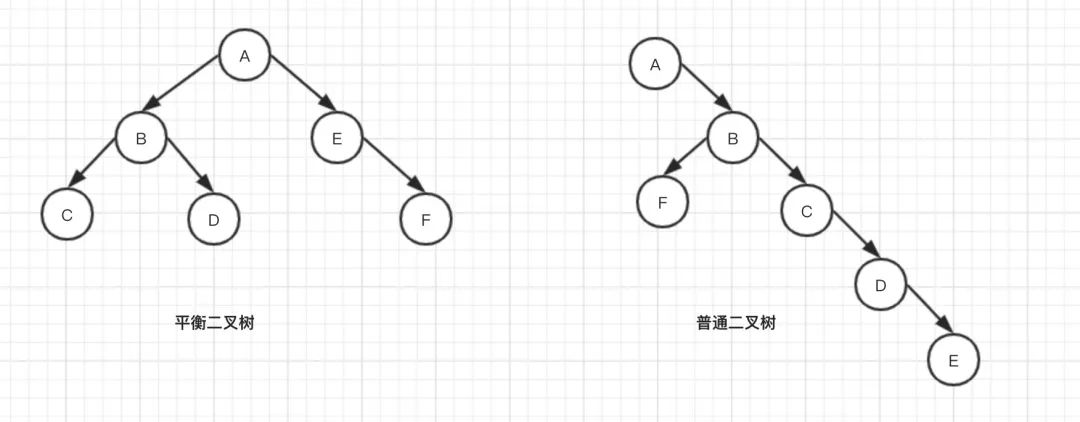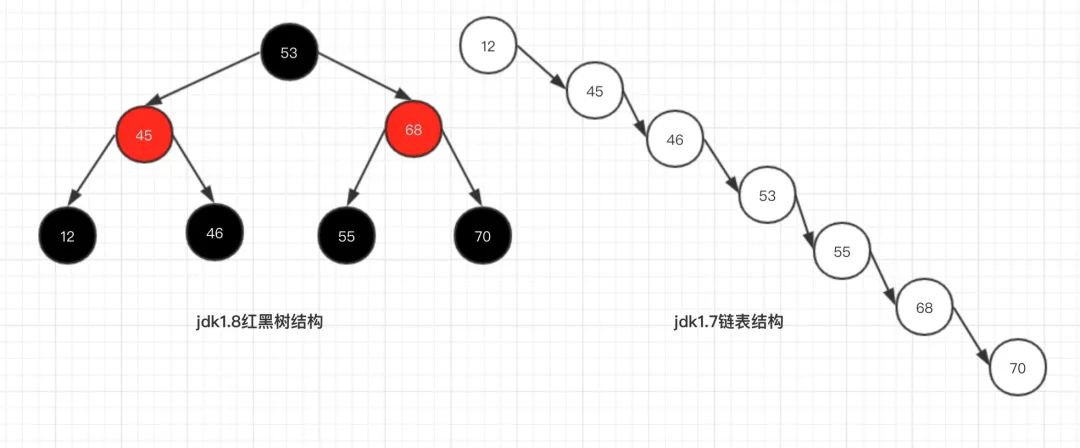# 面试官问JDK7和JDK8的HashMap不一样在哪里？我懵了

nill0705

0收藏

JDK7 的HashMap``````static final int DEFAULT_INITIAL_CAPACITY = 16;
static final float DEFAULT_LOAD_FACTOR = 0.75F;
int threshold;

static class Entry<K, V> implements java.util.Map.Entry<K, V> {
final K key;
V value;
HashMap.Entry<K, V> next;
int hash;

Entry(int var1, K var2, V var3, HashMap.Entry<K, V> var4) {
this.value = var3;
this.next = var4;
this.key = var2;
this.hash = var1;
}``````

CAPACITY: 当前数组容量，始终保持 2^n，可以扩容，扩容后数组大小为当前的 2 倍

JDK8 的HashMap

Java8 对 HashMap 进行了一些修改，最大的不同就是利用了红黑树，所以其由 数组+链表+红黑 树组成。`````` public HashMap(int initialCapacity, float loadFactor) {
if (initialCapacity < 0)
throw new IllegalArgumentException("Illegal initial capacity: " +
initialCapacity);
if (initialCapacity > MAXIMUM_CAPACITY)
initialCapacity = MAXIMUM_CAPACITY;
throw new IllegalArgumentException("Illegal load factor: " +
this.threshold = tableSizeFor(initialCapacity);
}

public HashMap(int initialCapacity) {
}

public HashMap() {
}``````

``````public HashMap(int initialCapacity, float loadFactor) {
if (initialCapacity < 0)
throw new IllegalArgumentException("Illegal initial capacity: " + initialCapacity);
if (initialCapacity > MAXIMUM_CAPACITY)
initialCapacity = MAXIMUM_CAPACITY;
threshold = initialCapacity;
init();
}

public HashMap(int initialCapacity) {
}

public HashMap() {
}``````●  1、每个节点要么是红色，要么是黑色，但根节点永远是黑色的；

●  2、每个红色节点的两个子节点一定都是黑色；

●  3、红色节点不能连续（也即是，红色节点的孩子和父亲都不能是红色）；

●  4、从任一节点到其子树中每个叶子节点的路径都包含相同数量的黑色节点；

●  5、所有的叶节点都是是黑色的（注意这里说叶子节点其实是上图中的 NIL 节点）；

【集合系列】- 红黑树实现分析

``````/**
* 红黑树的插入操作
*/
final TreeNode<K,V> putTreeVal(HashMap<K,V> map, Node<K,V>[] tab,
int h, K k, V v) {
Class<?> kc = null;
boolean searched = false;
TreeNode<K,V> root = (parent != null) ? root() : this;
for (TreeNode<K,V> p = root;;) {
//dir:遍历的方向， ph:p节点的hash值
int dir, ph; K pk;
//红黑树是根据hash值来判断大小
// -1:左孩子方向 1:右孩子方向
if ((ph = p.hash) > h)
dir = -1;
else if (ph < h)
dir = 1;
//如果key存在的话就直接返回当前节点
else if ((pk = p.key) == k || (k != null && k.equals(pk)))
return p;
//如果当前插入的类型和正在比较的节点的Key是Comparable的话，就直接通过此接口比较
else if ((kc == null &&
(kc = comparableClassFor(k)) == null) ||
(dir = compareComparables(kc, k, pk)) == 0) {
if (!searched) {
TreeNode<K,V> q, ch;
searched = true;
//尝试在p的左子树或者右子树中找到了目标元素
if (((ch = p.left) != null &&
(q = ch.find(h, k, kc)) != null) ||
((ch = p.right) != null &&
(q = ch.find(h, k, kc)) != null))
return q;
}
//获取遍历的方向
dir = tieBreakOrder(k, pk);
}
//上面的所有if-else判断都是在判断下一次进行遍历的方向，即dir
TreeNode<K,V> xp = p;
//当下面的if判断进去之后就代表找到了目标操作元素,即xp
if ((p = (dir <= 0) ? p.left : p.right) == null) {
Node<K,V> xpn = xp.next;
//插入新的元素
TreeNode<K,V> x = map.newTreeNode(h, k, v, xpn);
if (dir <= 0)
xp.left = x;
else
xp.right = x;
//因为TreeNode今后可能退化成链表，在这里需要维护链表的next属性
xp.next = x;
//完成节点插入操作
x.parent = x.prev = xp;
if (xpn != null)
((TreeNode<K,V>)xpn).prev = x;
//插入操作完成之后就要进行一定的调整操作了
moveRootToFront(tab, balanceInsertion(root, x));
return null;
}
}
}``````●  红黑树查询：其访问性能近似于折半查找，时间复杂度O(logn)；

●  链表查询：这种情况下，需要遍历全部元素才行，时间复杂度O(n)；帖子
视频
声望
粉丝
社区精华内容